Important: Please read the installation page for details about how to install the toolboxes. $\newcommand{\dotp}{\langle #1, #2 \rangle}$ $\newcommand{\enscond}{\lbrace #1, #2 \rbrace}$ $\newcommand{\pd}{ \frac{ \partial #1}{\partial #2} }$ $\newcommand{\umin}{\underset{#1}{\min}\;}$ $\newcommand{\umax}{\underset{#1}{\max}\;}$ $\newcommand{\umin}{\underset{#1}{\min}\;}$ $\newcommand{\uargmin}{\underset{#1}{argmin}\;}$ $\newcommand{\norm}{\|#1\|}$ $\newcommand{\abs}{\left|#1\right|}$ $\newcommand{\choice}{ \left\{ \begin{array}{l} #1 \end{array} \right. }$ $\newcommand{\pa}{\left(#1\right)}$ $\newcommand{\diag}{{diag}\left( #1 \right)}$ $\newcommand{\qandq}{\quad\text{and}\quad}$ $\newcommand{\qwhereq}{\quad\text{where}\quad}$ $\newcommand{\qifq}{ \quad \text{if} \quad }$ $\newcommand{\qarrq}{ \quad \Longrightarrow \quad }$ $\newcommand{\ZZ}{\mathbb{Z}}$ $\newcommand{\CC}{\mathbb{C}}$ $\newcommand{\RR}{\mathbb{R}}$ $\newcommand{\EE}{\mathbb{E}}$ $\newcommand{\Zz}{\mathcal{Z}}$ $\newcommand{\Ww}{\mathcal{W}}$ $\newcommand{\Vv}{\mathcal{V}}$ $\newcommand{\Nn}{\mathcal{N}}$ $\newcommand{\NN}{\mathcal{N}}$ $\newcommand{\Hh}{\mathcal{H}}$ $\newcommand{\Bb}{\mathcal{B}}$ $\newcommand{\Ee}{\mathcal{E}}$ $\newcommand{\Cc}{\mathcal{C}}$ $\newcommand{\Gg}{\mathcal{G}}$ $\newcommand{\Ss}{\mathcal{S}}$ $\newcommand{\Pp}{\mathcal{P}}$ $\newcommand{\Ff}{\mathcal{F}}$ $\newcommand{\Xx}{\mathcal{X}}$ $\newcommand{\Mm}{\mathcal{M}}$ $\newcommand{\Ii}{\mathcal{I}}$ $\newcommand{\Dd}{\mathcal{D}}$ $\newcommand{\Ll}{\mathcal{L}}$ $\newcommand{\Tt}{\mathcal{T}}$ $\newcommand{\si}{\sigma}$ $\newcommand{\al}{\alpha}$ $\newcommand{\la}{\lambda}$ $\newcommand{\ga}{\gamma}$ $\newcommand{\Ga}{\Gamma}$ $\newcommand{\La}{\Lambda}$ $\newcommand{\si}{\sigma}$ $\newcommand{\Si}{\Sigma}$ $\newcommand{\be}{\beta}$ $\newcommand{\de}{\delta}$ $\newcommand{\De}{\Delta}$ $\newcommand{\phi}{\varphi}$ $\newcommand{\th}{\theta}$ $\newcommand{\om}{\omega}$ $\newcommand{\Om}{\Omega}$

This tour explores the use of the conjugate gradient method for the solution of large scale symmetric linear systems.

In :
addpath('toolbox_signal')


## Conjugate Gradient to Solve Symmetric Linear Systems¶

The conjugate gradient method is an iterative method that is taylored to solve large symmetric linear systems $Ax=b$.

We first give an example using a full explicit matrix $A$, but one should keep in mind that this method is efficient especially when the matrix $A$ is sparse or more generally when it is fast to apply $A$ to a vector. This is usually the case in image processing, where $A$ is often composed of convolution, fast transform (wavelet, fourier) or diagonal operator (e.g. for inpainting).

One initializes the CG method as $$x_0 \in \RR^N, \quad r_0 = b - x_0, \quad p_0 = r_0$$ The iterations of the method reads $$\choice{ \alpha_k = \frac{ \dotp{r_k}{r_k} }{ \dotp{p_k}{A p_k} } \\ x_{k+1} = x_k + \alpha_k p_k \\ r_{k+1} = r_k - \alpha_k A p_k \\ \beta_k = \frac{ \dotp{r_{k+1}}{r_{k+1}} }{ \dotp{r_k}{r_k} } \\ p_{k+1} = r_k + \beta_k p_k }$$

Note that one has $r_k = b - Ax_k$ which is the residual at iteration $k$. One can thus stop the method when $\norm{r_k}$ is smaller than some user-defined threshold.

Dimension of the problem.

In :
n = 500;


Matrix $A$ of the linear system. We use here a random positive symmetric matrix and shift its diagonal to make it well conditionned.

In :
A = randn(n);
A = A*A' + .1*eye(n);


Right hand side of the linear system. We use here a random vector.

In :
b = randn(n,1);


Canonical inner product in $\RR^N$.

In :
dotp = @(a,b)sum(a(:).*b(:));


Exercise 1

Implement the conjugate gradient method, and monitor the decay of the energy $\norm{r_k}=\norm{Ax_k-b}$.

In :
exo1()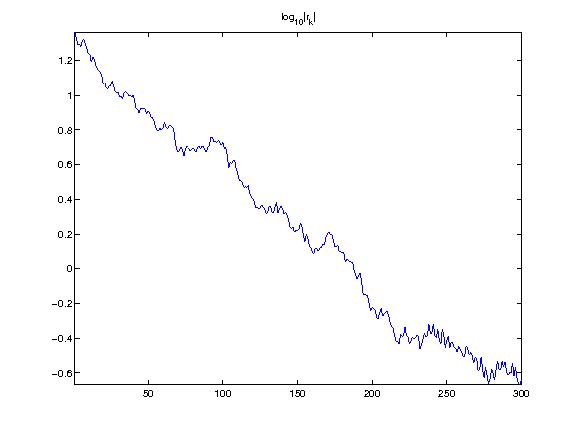In :
%% Insert your code here.


## Gradient and Divergence of Images¶

Local differential operators like gradient, divergence and laplacian are the building blocks for variational image processing.

Load an image $g \in \RR^N$ of $N=n \times n$ pixels.

In :
n = 256;


Display it.

In :
clf;
imageplot(g);For continuous functions, the gradient reads $$\nabla g(x) = \pa{ \pd{g(x)}{x_1}, \pd{g(x)}{x_2} } \in \RR^2.$$ (note that here, the variable $x$ denotes the 2-D spacial position).

We discretize this differential operator using first order finite differences. $$(\nabla g)_i = ( g_{i_1,i_2}-g_{i_1-1,i_2}, g_{i_1,i_2}-g_{i_1,i_2-1} ) \in \RR^2.$$ Note that for simplity we use periodic boundary conditions.

Compute its gradient, using finite differences.

In :
s = [n 1:n-1];


One thus has $\nabla : \RR^N \mapsto \RR^{N \times 2}.$

In :
v = grad(g);


One can display each of its components.

In :
clf;
imageplot(v(:,:,1), 'd/dx', 1,2,1);
imageplot(v(:,:,2), 'd/dy', 1,2,2);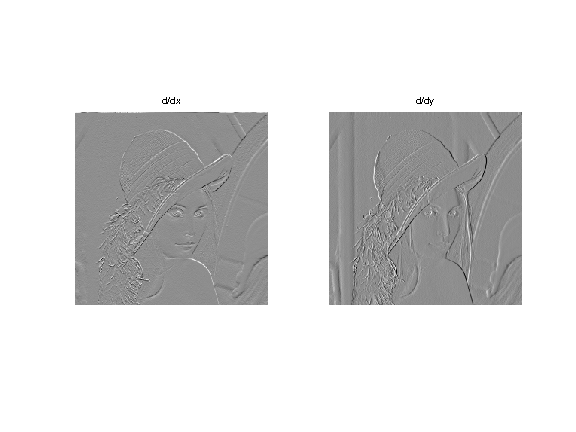One can also display it using a color image.

In :
clf;
imageplot(v);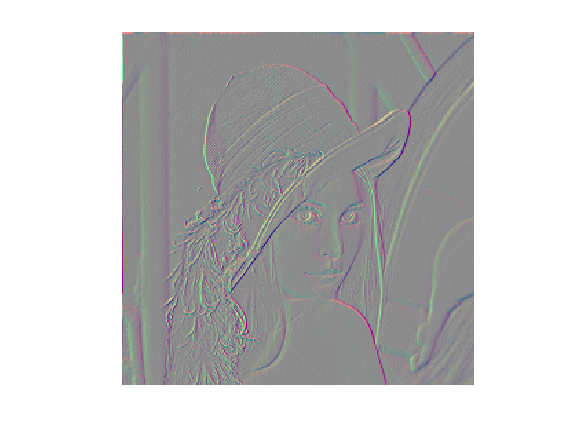One can display its magnitude $\norm{\nabla g(x)}$, which is large near edges.

In :
clf;
imageplot( sqrt( sum3(v.^2,3) ) );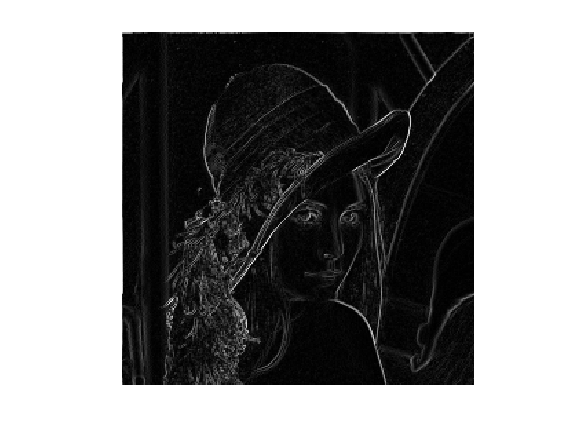The divergence operator maps vector field to images. For continuous vector fields $v(x) \in \RR^2$, it is defined as $$\text{div}(v)(x) = \pd{v_1(x)}{x_1} + \pd{v_2(x)}{x_2} \in \RR.$$ (note that here, the variable $x$ denotes the 2-D spacial position). It is minus the adjoint of the gadient, i.e. $\text{div} = - \nabla^*$.

It is discretized, for $v=(v^1,v^2)$ as $$\text{div}(v)_i = v^1_{i_1+1,i_2} - v^1_{i_1,i_2} + v^2_{i_1,i_2+1} - v^2_{i_1,i_2} .$$

In :
t = [2:n 1];
div = @(v)v(t,:,1)-v(:,:,1) + v(:,t,2)-v(:,:,2);


The Laplacian operatore is defined as $\Delta=\text{div} \circ \nabla = -\nabla^* \circ \nabla$. It is thus a negative symmetric operator.

In :
delta = @(f)div(grad(f));


Display $\Delta f_0$.

In :
clf;
imageplot(delta(g));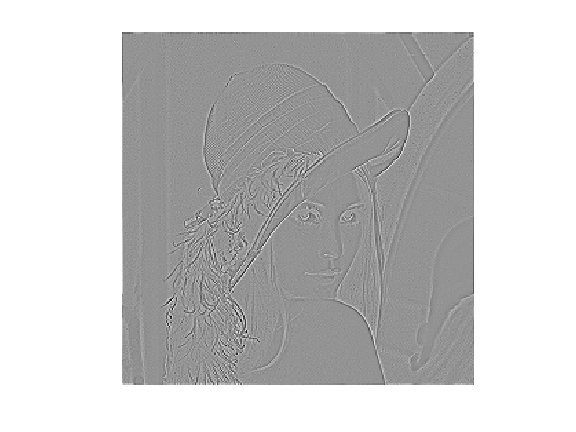Check that the relation $\norm{\nabla f} = - \dotp{\Delta f}{f}.$

In :
dotp = @(a,b)sum(a(:).*b(:));

Should be 0: 000


## Conjugate Gradient for Lagrangian Inpainting¶

We consider here the inpainting problem, which corresponds to the interpolation of missing data in the image.

We define a binary mask $M \in \RR^N$ where $M_i=0$ if the pixel indexed by $i$ is missing, and $M_i=1$ otherwise. We consider here random missing pixel, and a large missing region in the upper left corner.

In :
M = rand(n)>.7;
w = 30;
M(end/4-w:end/4+w,end/4-w:end/4+w) = 0;


Define the degradation operator $\Phi : \RR^N \rightarrow \RR^N$, that corresponds to the masking with $M$, i.e. a diagonal operator $$\Phi = \text{diag}_i(M_i).$$

In :
Phi = @(x)M.*x;


Compute the observations $y = \Phi(x)$ with damaged pixels.

In :
y = Phi(g);


Display the observed image.

In :
clf;
imageplot(y);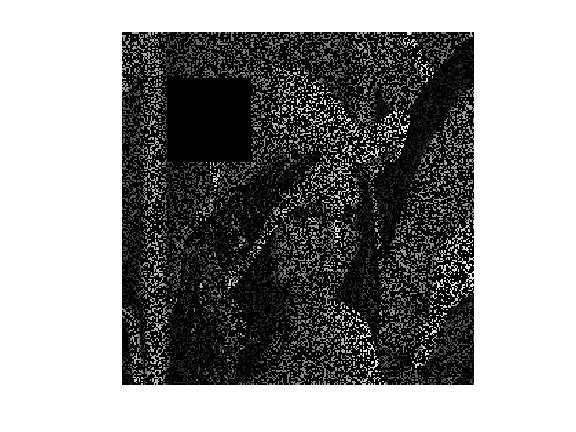To perform the recovery of an image from the damaged observations $y$, we aim at finding an image $x$ that agrees as much with the measurements, i.e. $\Phi x \approx y$, but at the same time is smooth. We measure the smoothness using the norm of the gradient $\norm{\nabla x}^2$, which corresponds to a discret Sobolev norm.

This leads us to consider the following quadratic minimization problem $$\umin{x \in \RR^N} F(x) = \norm{y-\Phi x}^2 + \la \norm{\nabla x}^2.$$

This problem as a unique solution if $\text{ker}(\Phi) \cap \text{ker}(\nabla) = \{0\}$. This condition holds in our case since $\text{ker}(\nabla)$ is the set of constant images.

The solution can be obtained by solving the following linear system $$A x = b \qwhereq \choice{ A = \Phi^*\Phi - \la \Delta, \\ b = \Phi^* y. }$$ Here we can remark that for the inpainting problem, $\Phi^*\Phi=\Phi$ and $\Phi^*y=y$.

The value of the parameter $\lambda$ should be small.

In :
lambda = .01;


Operator to invert.

In :
A = @(x)Phi(x) - lambda*delta(x);


Right hand side of the linear system is $$b = \Phi^*(y) = y.$$

In :
b = y;


Exercise 2

Implement the conjugate gradient method, and monitor the decay of the energy $F(x_k)$.

In :
exo2()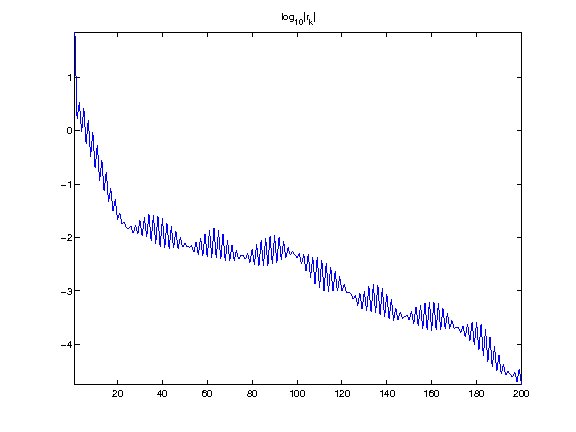In :
%% Insert your code here.


Display the result.

In :
clf;
imageplot(clamp(x));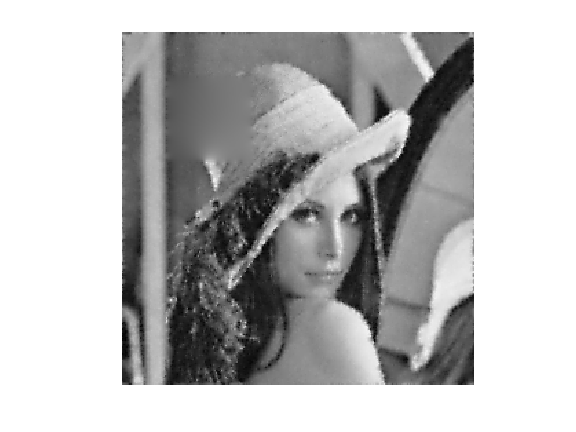## Conjugate Gradient for Constrained Inpainting¶

Since there is no noise perturbating the observation, it makes sense to use a $\lambda$ that is as small as possible.

When $\lambda \rightarrow 0$, the problem becomes $$\umin{\Phi x = y} \norm{\nabla x}^2.$$ This problem as a unique solution if $y\in \text{Im}(\Phi)$ and if $\text{ker}(\Phi) \cap \text{ker}(\nabla) = \{0\}$. This condition holds in our case, as we have already seen.

Introducing Lagrange multiplizers $u \in \RR^N$, this problem is equivalent to the resolution of the following couple of linear equations $$\choice{ -\Delta x + \Phi^* u = 0, \\ \Phi x = y. }$$

This corresponds to a single linear system over $z = (x,u) \in \RR^{N}$ \times \RR^N \sim \RR^{N \times 2}$$$A z = b \qwhereq A = \begin{pmatrix} -\Delta & \Phi^* \\ \Phi & 0 \end{pmatrix} \qandq b = \begin{pmatrix} 0 \\ y \end{pmatrix}$$ Define the operator$A$. Note that$x$is encoded in |z(:,:,1)| and$u$in |z(:,:,2)|. In : A = @(z)cat(3, -delta(z(:,:,1)) + Phi(z(:,:,2)), Phi(z(:,:,1)) );  Define the right hand side$b$. In : b = cat(3, zeros(n), y);  Exercise 3 Implement the conjugate gradient method, and monitor the decay of the energy$F(x_k)) = \norm{\nabla x_k}$and the constraint$C(x_k) = \norm{y-\Phi x_k}^2\$. Important: be carefull at the initialization of the method.

In :
exo3()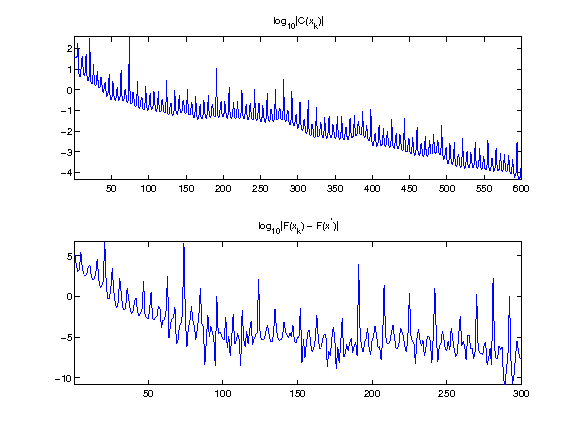In :
%% Insert your code here.


Display the result.

In :
clf;
imageplot(x);